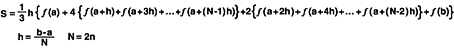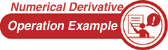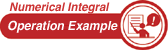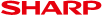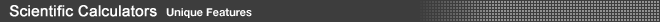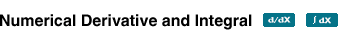Simply enter numerical values following the interactive on-screen instructions, and you can find the answer easily of derivative and integral calculations. You don't have to re-enter the formula, and the calculation can be repeated as many times as needed.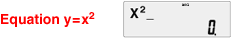1 Numerical Derivative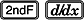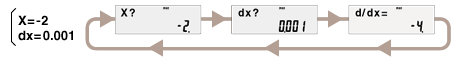You can calculate the same derivative calculation repeatedly without having to input the formula again.
2 Numerical Integral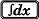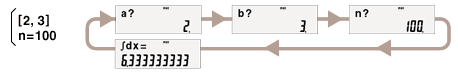You can calculate the same integral calculation repeatedly without having to input the formula again.
 Note: These derivative/integral calculations are performed based on the following equations. • Derivative calculation: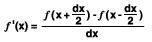• Integral calculation (Simpson's rule):